Updating search results...

# 9 Results

View
Selected filters:
• Aubrey McKelvey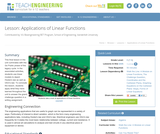Educational Use
Rating
0.0 stars

This final lesson in the unit culminates with the Go Public phase of the legacy cycle. In the associated activities, students use linear models to depict Hooke's law as well as Ohm's law. To conclude the lesson, students apply they have learned throughout the unit to answer the grand challenge question in a writing assignment.

Subject:
Engineering
Mathematics
Algebra
Material Type:
Lesson Plan
Provider:
TeachEngineering
Provider Set:
TeachEngineering
Author:
Aubrey McKelvey
09/18/2014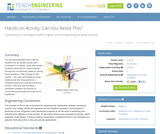Educational Use
Rating
0.0 stars

This lab demonstrates Ohm's law as students set up simple circuits each composed of a battery, lamp and resistor. Students calculate the current flowing through the circuits they create by solving linear equations. After solving for the current, I, for each set resistance value, students plot the three points on a Cartesian plane and note the line that is formed. They also see the direct correlation between the amount of current flowing through the lamp and its brightness.

Subject:
Engineering
Physics
Material Type:
Activity/Lab
Provider:
TeachEngineering
Provider Set:
TeachEngineering
Author:
Aubrey McKelvey
09/18/2014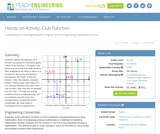Educational Use
Rating
0.0 stars

Students explore the definition of a function by playing an interactive game called "Club Function." The goal of the game is to be in the club! With students each assigned to be either a zebra or a rhinoceros, they group themselves according to the "rules" of the club function. After two minutes, students freeze in their groups, and if they are not correctly following the rules of the club function, then they are not allowed into the "club." Through this activity students come to understand that one x-coordinate can only have one corresponding y-coordinate while y-coordinates can have many x-coordinates that correspond to it.

Subject:
Engineering
Mathematics
Material Type:
Activity/Lab
Provider:
TeachEngineering
Provider Set:
TeachEngineering
Author:
Aubrey McKelvey
09/18/2014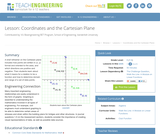Educational Use
Rating
0.0 stars

A brief refresher on the Cartesian plane includes how points are written in (x, y) format and oriented to the axes, and which directions are positive and negative. Then students learn about what it means for a relation to be a function and how to determine domain and range of a set of data points.

Subject:
Engineering
Mathematics
Algebra
Material Type:
Lesson Plan
Provider:
TeachEngineering
Provider Set:
TeachEngineering
Author:
Aubrey McKelvey
09/18/2014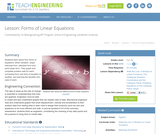Educational Use
Rating
0.0 stars

Students learn about four forms of equations: direct variation, slope-intercept form, standard form and point-slope form. They graph and complete problem sets for each, converting from one form of equation to another, and learning the benefits and uses of each.

Subject:
Engineering
Mathematics
Algebra
Material Type:
Lesson Plan
Provider:
TeachEngineering
Provider Set:
TeachEngineering
Author:
Aubrey McKelvey
09/18/2014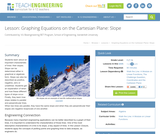Educational Use
Rating
0.0 stars

Students learn about an important characteristic of lines: their slopes. Slope can be determined either in graphical or algebraic form. Slope can also be described as positive, negative, zero or undefined. Students get an explanation of when and how these different types of slope occur. Finally, they learn how slope relates to parallel and perpendicular lines. When two lines are parallel, they have the same slope and when they are perpendicular their slopes are negative reciprocals of one another.

Subject:
Engineering
Mathematics
Algebra
Material Type:
Lesson Plan
Provider:
TeachEngineering
Provider Set:
TeachEngineering
Author:
Aubrey Mckelvey
09/18/2014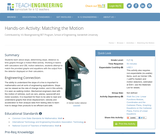Educational Use
Rating
0.0 stars

Students learn about slope, determining slope, distance vs. time graphs through a motion-filled activity. Working in teams with calculators and CBL motion detectors, students attempt to match the provided graphs and equations with the output from the detector displayed on their calculators.

Subject:
Engineering
Mathematics
Physics
Material Type:
Activity/Lab
Provider:
TeachEngineering
Provider Set:
TeachEngineering
Author:
Aubrey McKelvey
09/18/2014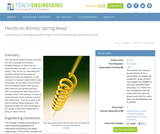Educational Use
Rating
0.0 stars

This lab demonstrates Hooke's Law with the use of springs and masses. Students attempt to determine the proportionality constant, or k-value, for a spring. They do this by calculating the change in length of the spring as different masses are added to it. The concept of a spring's elastic limit is also introduced, and the students test to makes sure the spring's elastic limit has not been reached during their lab tests. After compiling their data, they attempt to find an average value of the spring's k-value by measuring the slopes between each of their data points. Then they apply what they've learned about springs to how engineers might use that knowledge in the design of a toy that enables kids to jump 2-3 feet in the air.

Subject:
Engineering
Life Science
Mathematics
Material Type:
Activity/Lab
Provider:
TeachEngineering
Provider Set:
TeachEngineering
Author:
Aubrey Mckelvey
09/18/2014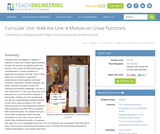Educational Use
Rating
0.0 stars

Prepared with pre-algebra or algebra 1 classes in mind, this module leads students through the process of graphing data and finding a line of best fit while exploring the characteristics of linear equations in algebraic and graphic formats. Then, these topics are connected to real-world experiences in which people use linear functions. During the module, students use these scientific concepts to solve the following hypothetical challenge: You are a new researcher in a lab, and your boss has just given you your first task to analyze a set of data. It being your first assignment, you ask an undergraduate student working in your lab to help you figure it out. She responds that you must determine what the data represents and then find an equation that models the data. You believe that you will be able to determine what the data represents on your own, but you ask for further help modeling the data. In response, she says she is not completely sure how to do it, but gives a list of equations that may fit the data. This module is built around the legacy cycle, a format that incorporates educational research feindings on how people best learn.

Subject:
Engineering
Life Science
Mathematics
Algebra
Material Type:
Unit of Study
Provider:
TeachEngineering
Provider Set:
TeachEngineering
Author:
Aubrey Mckelvey